# Sequential Circuits

Advertisements

The combinational circuit does not use any memory. Hence the previous state of input does not have any effect on the present state of the circuit. But sequential circuit has memory so output can vary based on input. This type of circuits uses previous input, output, clock and a memory element.

## Block diagram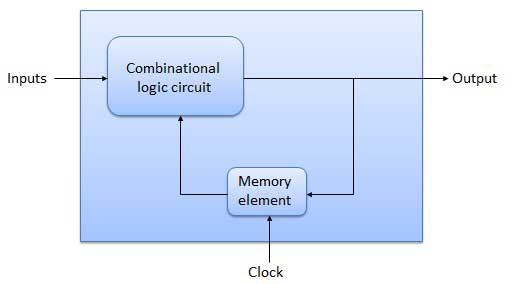## Flip Flop

Flip flop is a sequential circuit which generally samples its inputs and changes its outputs only at particular instants of time and not continuously. Flip flop is said to be edge sensitive or edge triggered rather than being level triggered like latches.

## S-R Flip Flop

It is basically S-R latch using NAND gates with an additional enable input. It is also called as level triggered SR-FF. For this, circuit in output will take place if and only if the enable input (E) is made active. In short this circuit will operate as an S-R latch if E = 1 but there is no change in the output if E = 0.

### Block Diagram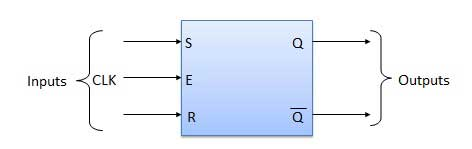### Circuit Diagram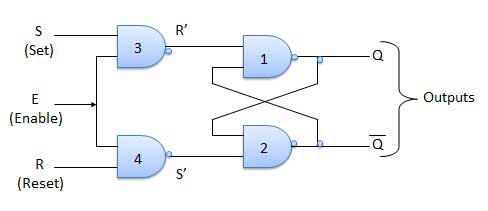### Truth Table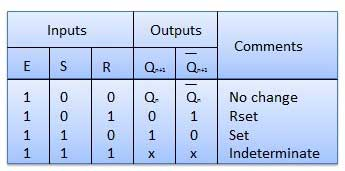### Operation

S.N. Condition Operation
1 S = R = 0 : No change

If S = R = 0 then output of NAND gates 3 and 4 are forced to become 1.

Hence R' and S' both will be equal to 1. Since S' and R' are the input of the basic S-R latch using NAND gates, there will be no change in the state of outputs.

2 S = 0, R = 1, E = 1

Since S = 0, output of NAND-3 i.e. R' = 1 and E = 1 the output of NAND-4 i.e. S' = 0.

Hence Qn+1 = 0 and Qn+1 bar = 1. This is reset condition.

3 S = 1, R = 0, E = 1

Output of NAND-3 i.e. R' = 0 and output of NAND-4 i.e. S' = 1.

Hence output of S-R NAND latch is Qn+1 = 1 and Qn+1 bar = 0. This is the reset condition.

4 S = 1, R = 1, E = 1

As S = 1, R = 1 and E = 1, the output of NAND gates 3 and 4 both are 0 i.e. S' = R' = 0.

Hence the Race condition will occur in the basic NAND latch.

## Master Slave JK Flip Flop

Master slave JK FF is a cascade of two S-R FF with feedback from the output of second to input of first. Master is a positive level triggered. But due to the presence of the inverter in the clock line, the slave will respond to the negative level. Hence when the clock = 1 (positive level) the master is active and the slave is inactive. Whereas when clock = 0 (low level) the slave is active and master is inactive.

### Circuit Diagram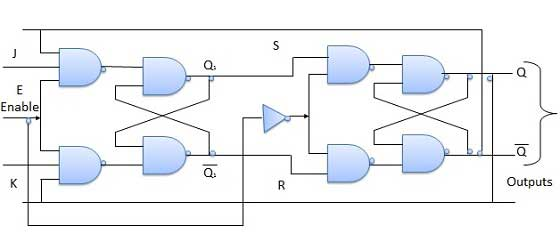### Truth Table### Operation

S.N. Condition Operation
1 J = K = 0 (No change)

When clock = 0, the slave becomes active and master is inactive. But since the S and R inputs have not changed, the slave outputs will also remain unchanged. Therefore outputs will not change if J = K =0.

2 J = 0 and K = 1 (Reset)

Clock = 1 − Master active, slave inactive. Therefore outputs of the master become Q1 = 0 and Q1 bar = 1. That means S = 0 and R =1.

Clock = 0 − Slave active, master inactive. Therefore outputs of the slave become Q = 0 and Q bar = 1.

Again clock = 1 − Master active, slave inactive. Therefore even with the changed outputs Q = 0 and Q bar = 1 fed back to master, its output will be Q1 = 0 and Q1 bar = 1. That means S = 0 and R = 1.

Hence with clock = 0 and slave becoming active the outputs of slave will remain Q = 0 and Q bar = 1. Thus we get a stable output from the Master slave.

3 J = 1 and K = 0 (Set)

Clock = 1 − Master active, slave inactive. Therefore outputs of the master become Q1 = 1 and Q1 bar = 0. That means S = 1 and R =0.

Clock = 0 − Slave active, master inactive. Therefore outputs of the slave become Q = 1 and Q bar = 0.

Again clock = 1 − then it can be shown that the outputs of the slave are stabilized to Q = 1 and Q bar = 0.

4 J = K = 1 (Toggle)

Clock = 1 − Master active, slave inactive. Outputs of master will toggle. So S and R also will be inverted.

Clock = 0 − Slave active, master inactive. Outputs of slave will toggle.

These changed output are returned back to the master inputs. But since clock = 0, the master is still inactive. So it does not respond to these changed outputs. This avoids the multiple toggling which leads to the race around condition. The master slave flip flop will avoid the race around condition.

## Delay Flip Flop / D Flip Flop

Delay Flip Flop or D Flip Flop is the simple gated S-R latch with a NAND inverter connected between S and R inputs. It has only one input. The input data is appearing at the output after some time. Due to this data delay between i/p and o/p, it is called delay flip flop. S and R will be the complements of each other due to NAND inverter. Hence S = R = 0 or S = R = 1, these input condition will never appear. This problem is avoid by SR = 00 and SR = 1 conditions.

### Block Diagram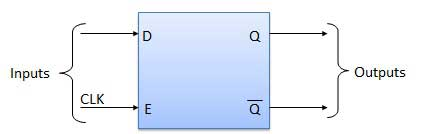### Circuit Diagram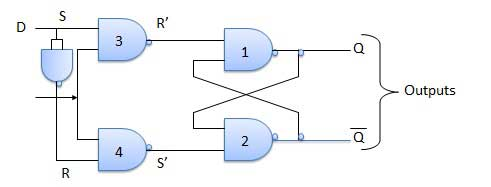### Truth Table### Operation

S.N. Condition Operation
1 E = 0

Latch is disabled. Hence no change in output.

2 E = 1 and D = 0

If E = 1 and D = 0 then S = 0 and R = 1. Hence irrespective of the present state, the next state is Qn+1 = 0 and Qn+1 bar = 1. This is the reset condition.

3 E = 1 and D = 1

If E = 1 and D = 1, then S = 1 and R = 0. This will set the latch and Qn+1 = 1 and Qn+1 bar = 0 irrespective of the present state.

## Toggle Flip Flop / T Flip Flop

Toggle flip flop is basically a JK flip flop with J and K terminals permanently connected together. It has only input denoted by T as shown in the Symbol Diagram. The symbol for positive edge triggered T flip flop is shown in the Block Diagram.

### Symbol Diagram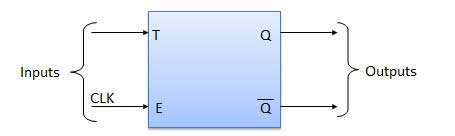### Block Diagram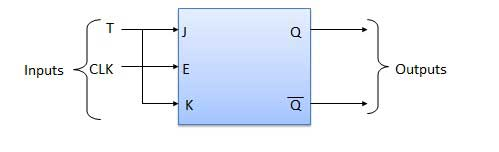### Truth Table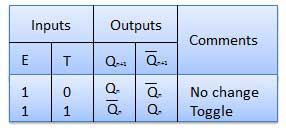### Operation

S.N. Condition Operation
1 T = 0, J = K = 0 The output Q and Q bar won't change
2 T = 1, J = K = 1 Output will toggle corresponding to every leading edge of clock signal.
Advertisements